# Basic electronics

28. Apr 2013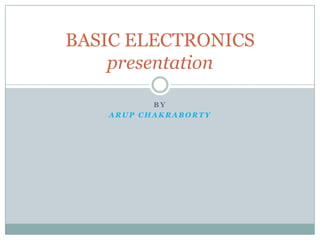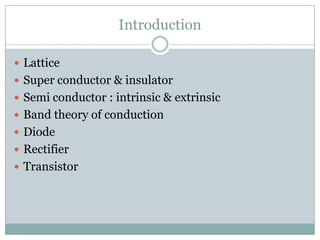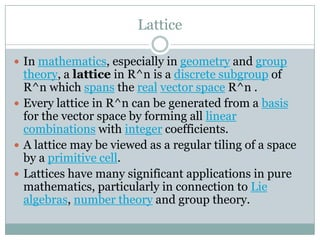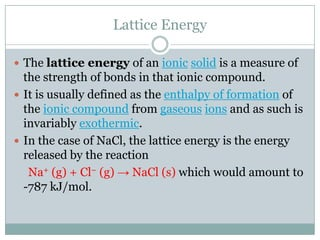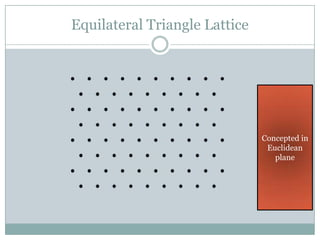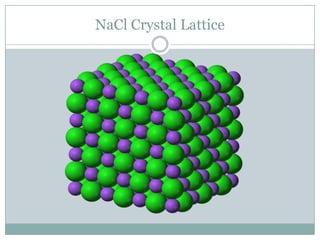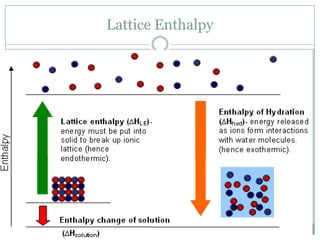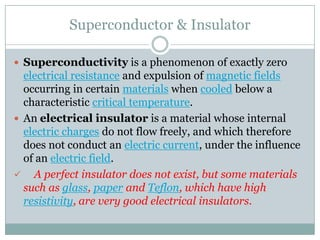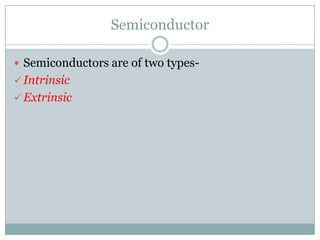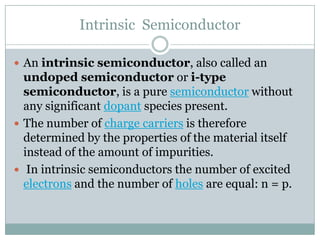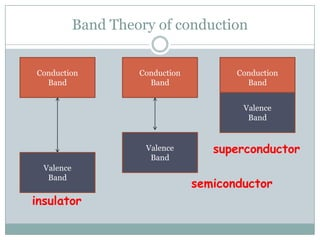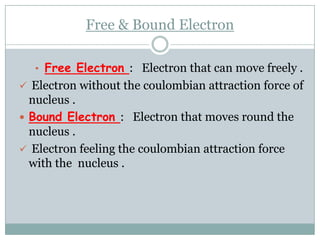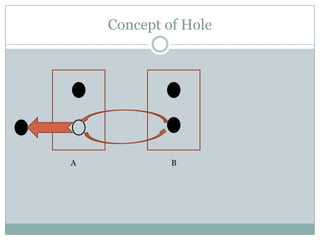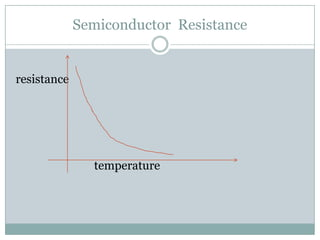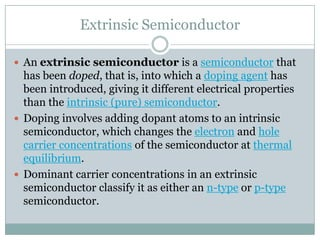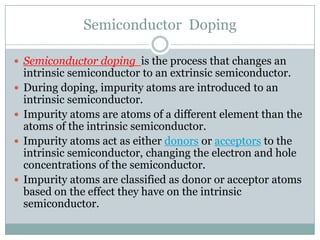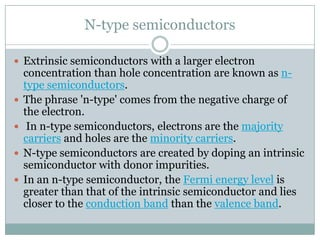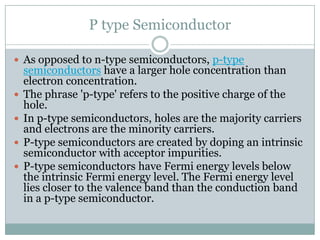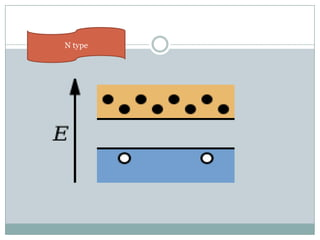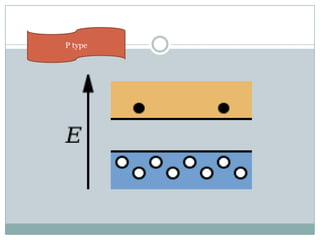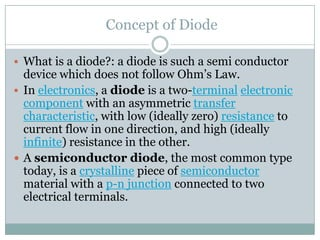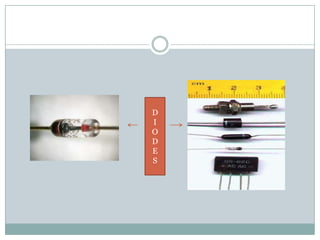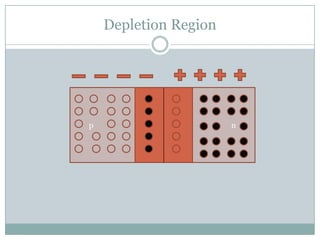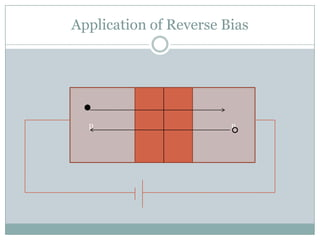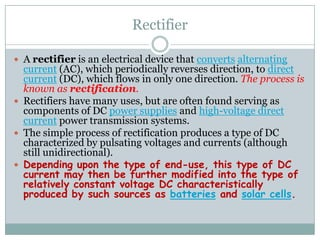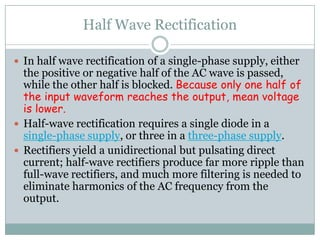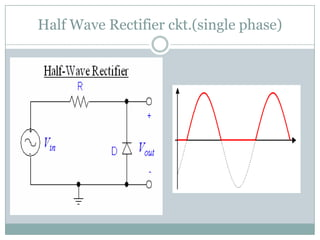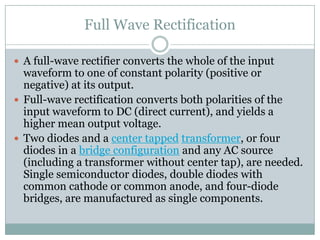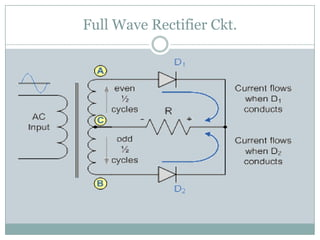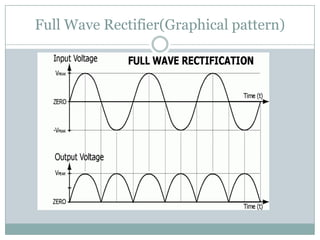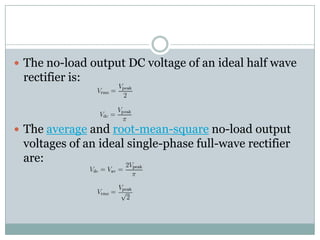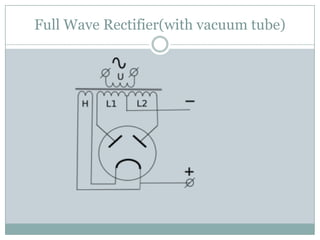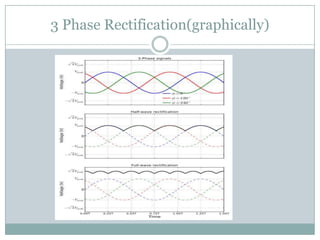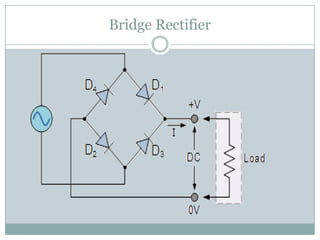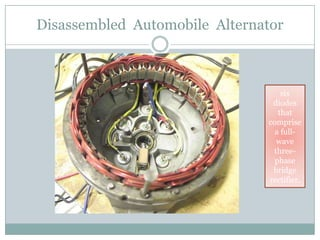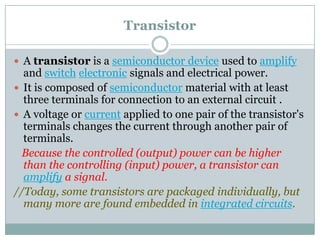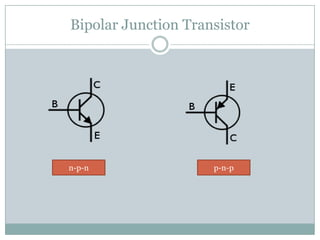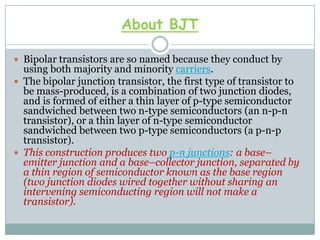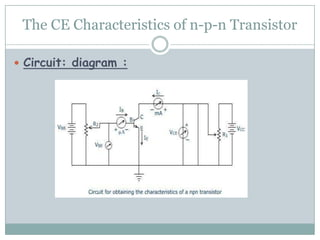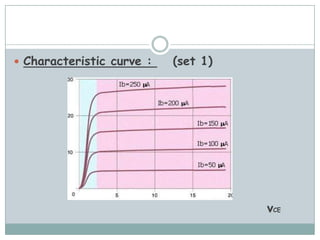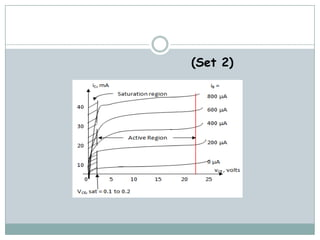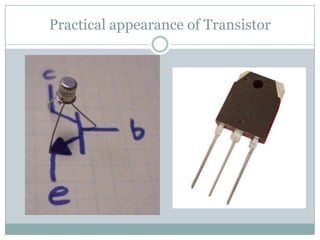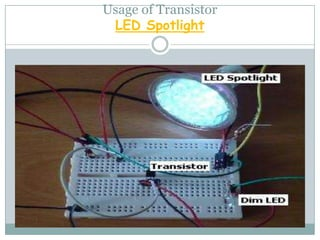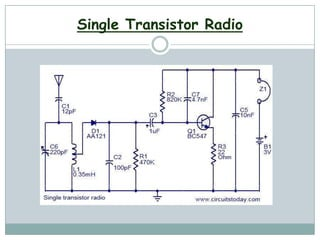1 von 46

### Basic electronics

• 1. B Y A R U P C H A K R A B O R T Y BASIC ELECTRONICS presentation
• 2. Introduction  Lattice  Super conductor & insulator  Semi conductor : intrinsic & extrinsic  Band theory of conduction  Diode  Rectifier  Transistor
• 3. Lattice  In mathematics, especially in geometry and group theory, a lattice in R^n is a discrete subgroup of R^n which spans the real vector space R^n .  Every lattice in R^n can be generated from a basis for the vector space by forming all linear combinations with integer coefficients.  A lattice may be viewed as a regular tiling of a space by a primitive cell.  Lattices have many significant applications in pure mathematics, particularly in connection to Lie algebras, number theory and group theory.
• 4. Lattice Energy  The lattice energy of an ionic solid is a measure of the strength of bonds in that ionic compound.  It is usually defined as the enthalpy of formation of the ionic compound from gaseous ions and as such is invariably exothermic.  In the case of NaCl, the lattice energy is the energy released by the reaction Na+ (g) + Cl− (g) → NaCl (s) which would amount to -787 kJ/mol.
• 5. Equilateral Triangle Lattice Concepted in Euclidean plane
• 6. NaCl Crystal Lattice
• 7. Lattice Enthalpy
• 8. Superconductor & Insulator  Superconductivity is a phenomenon of exactly zero electrical resistance and expulsion of magnetic fields occurring in certain materials when cooled below a characteristic critical temperature.  An electrical insulator is a material whose internal electric charges do not flow freely, and which therefore does not conduct an electric current, under the influence of an electric field.  A perfect insulator does not exist, but some materials such as glass, paper and Teflon, which have high resistivity, are very good electrical insulators.
• 9. Semiconductor  Semiconductors are of two types-  Intrinsic  Extrinsic
• 10. Intrinsic Semiconductor  An intrinsic semiconductor, also called an undoped semiconductor or i-type semiconductor, is a pure semiconductor without any significant dopant species present.  The number of charge carriers is therefore determined by the properties of the material itself instead of the amount of impurities.  In intrinsic semiconductors the number of excited electrons and the number of holes are equal: n = p.
• 11. Band Theory of conduction superconductor semiconductor insulator Conduction Band Conduction Band Conduction Band Valence Band Valence Band Valence Band
• 12. Free & Bound Electron • Free Electron : Electron that can move freely .  Electron without the coulombian attraction force of nucleus .  Bound Electron : Electron that moves round the nucleus .  Electron feeling the coulombian attraction force with the nucleus .
• 13. Concept of Hole A B
• 14. Semiconductor Resistance resistance temperature
• 15. Extrinsic Semiconductor  An extrinsic semiconductor is a semiconductor that has been doped, that is, into which a doping agent has been introduced, giving it different electrical properties than the intrinsic (pure) semiconductor.  Doping involves adding dopant atoms to an intrinsic semiconductor, which changes the electron and hole carrier concentrations of the semiconductor at thermal equilibrium.  Dominant carrier concentrations in an extrinsic semiconductor classify it as either an n-type or p-type semiconductor.
• 16. Semiconductor Doping  Semiconductor doping is the process that changes an intrinsic semiconductor to an extrinsic semiconductor.  During doping, impurity atoms are introduced to an intrinsic semiconductor.  Impurity atoms are atoms of a different element than the atoms of the intrinsic semiconductor.  Impurity atoms act as either donors or acceptors to the intrinsic semiconductor, changing the electron and hole concentrations of the semiconductor.  Impurity atoms are classified as donor or acceptor atoms based on the effect they have on the intrinsic semiconductor.
• 17.  The two types of extrinsic semiconductor N-type semiconductors P-type semiconductors
• 18. N-type semiconductors  Extrinsic semiconductors with a larger electron concentration than hole concentration are known as n- type semiconductors.  The phrase 'n-type' comes from the negative charge of the electron.  In n-type semiconductors, electrons are the majority carriers and holes are the minority carriers.  N-type semiconductors are created by doping an intrinsic semiconductor with donor impurities.  In an n-type semiconductor, the Fermi energy level is greater than that of the intrinsic semiconductor and lies closer to the conduction band than the valence band.
• 19. P type Semiconductor  As opposed to n-type semiconductors, p-type semiconductors have a larger hole concentration than electron concentration.  The phrase 'p-type' refers to the positive charge of the hole.  In p-type semiconductors, holes are the majority carriers and electrons are the minority carriers.  P-type semiconductors are created by doping an intrinsic semiconductor with acceptor impurities.  P-type semiconductors have Fermi energy levels below the intrinsic Fermi energy level. The Fermi energy level lies closer to the valence band than the conduction band in a p-type semiconductor.
• 20. N type
• 21. P type
• 22. Concept of Diode  What is a diode?: a diode is such a semi conductor device which does not follow Ohm’s Law.  In electronics, a diode is a two-terminal electronic component with an asymmetric transfer characteristic, with low (ideally zero) resistance to current flow in one direction, and high (ideally infinite) resistance in the other.  A semiconductor diode, the most common type today, is a crystalline piece of semiconductor material with a p-n junction connected to two electrical terminals.
• 23. D I O D E S
• 24. Depletion Region p n
• 25. Application of Reverse Bias p n
• 26. Rectifier  A rectifier is an electrical device that converts alternating current (AC), which periodically reverses direction, to direct current (DC), which flows in only one direction. The process is known as rectification.  Rectifiers have many uses, but are often found serving as components of DC power supplies and high-voltage direct current power transmission systems.  The simple process of rectification produces a type of DC characterized by pulsating voltages and currents (although still unidirectional).  Depending upon the type of end-use, this type of DC current may then be further modified into the type of relatively constant voltage DC characteristically produced by such sources as batteries and solar cells.
• 27. Half Wave Rectification  In half wave rectification of a single-phase supply, either the positive or negative half of the AC wave is passed, while the other half is blocked. Because only one half of the input waveform reaches the output, mean voltage is lower.  Half-wave rectification requires a single diode in a single-phase supply, or three in a three-phase supply.  Rectifiers yield a unidirectional but pulsating direct current; half-wave rectifiers produce far more ripple than full-wave rectifiers, and much more filtering is needed to eliminate harmonics of the AC frequency from the output.
• 28. Half Wave Rectifier ckt.(single phase)
• 29. Full Wave Rectification  A full-wave rectifier converts the whole of the input waveform to one of constant polarity (positive or negative) at its output.  Full-wave rectification converts both polarities of the input waveform to DC (direct current), and yields a higher mean output voltage.  Two diodes and a center tapped transformer, or four diodes in a bridge configuration and any AC source (including a transformer without center tap), are needed. Single semiconductor diodes, double diodes with common cathode or common anode, and four-diode bridges, are manufactured as single components.
• 30. Full Wave Rectifier Ckt.
• 31. Full Wave Rectifier(Graphical pattern)
• 32.  The no-load output DC voltage of an ideal half wave rectifier is:  The average and root-mean-square no-load output voltages of an ideal single-phase full-wave rectifier are:
• 33. Full Wave Rectifier(with vacuum tube)
• 34. 3 Phase Rectification(graphically)
• 35. Bridge Rectifier
• 36. Disassembled Automobile Alternator six diodes that comprise a full- wave three- phase bridge rectifier.
• 37. Transistor  A transistor is a semiconductor device used to amplify and switch electronic signals and electrical power.  It is composed of semiconductor material with at least three terminals for connection to an external circuit .  A voltage or current applied to one pair of the transistor's terminals changes the current through another pair of terminals. Because the controlled (output) power can be higher than the controlling (input) power, a transistor can amplify a signal. //Today, some transistors are packaged individually, but many more are found embedded in integrated circuits.
• 38. Bipolar Junction Transistor n-p-n p-n-p
• 39. About BJT  Bipolar transistors are so named because they conduct by using both majority and minority carriers.  The bipolar junction transistor, the first type of transistor to be mass-produced, is a combination of two junction diodes, and is formed of either a thin layer of p-type semiconductor sandwiched between two n-type semiconductors (an n-p-n transistor), or a thin layer of n-type semiconductor sandwiched between two p-type semiconductors (a p-n-p transistor).  This construction produces two p-n junctions: a base– emitter junction and a base–collector junction, separated by a thin region of semiconductor known as the base region (two junction diodes wired together without sharing an intervening semiconducting region will not make a transistor).
• 40. The CE Characteristics of n-p-n Transistor  Circuit: diagram :
• 41.  Characteristic curve : (set 1) IC vCE
• 42. (Set 2)
• 43. Practical appearance of Transistor
• 44. Usage of Transistor LED Spotlight